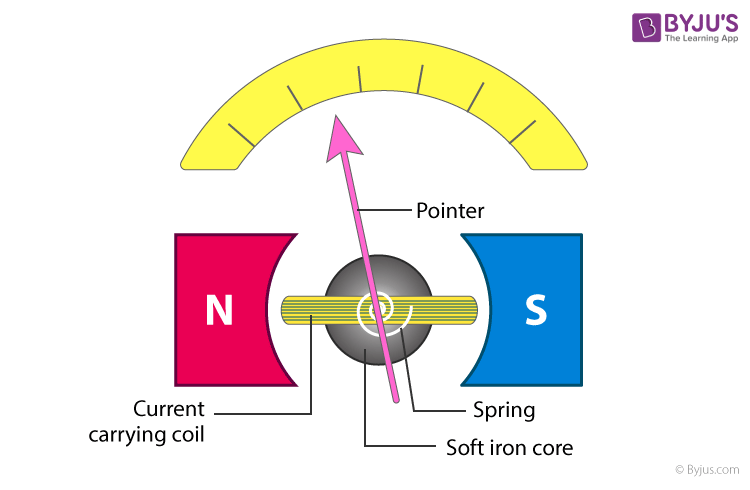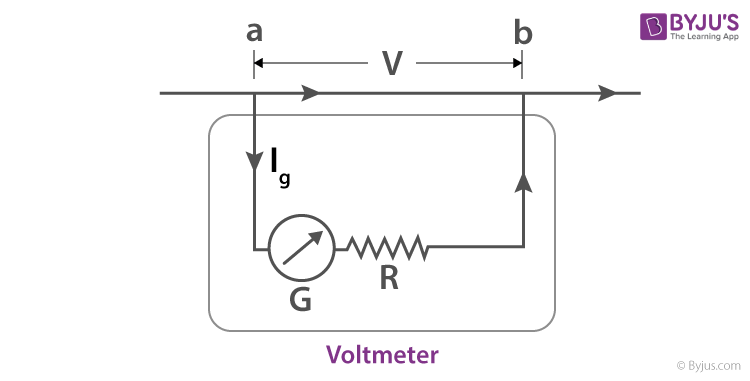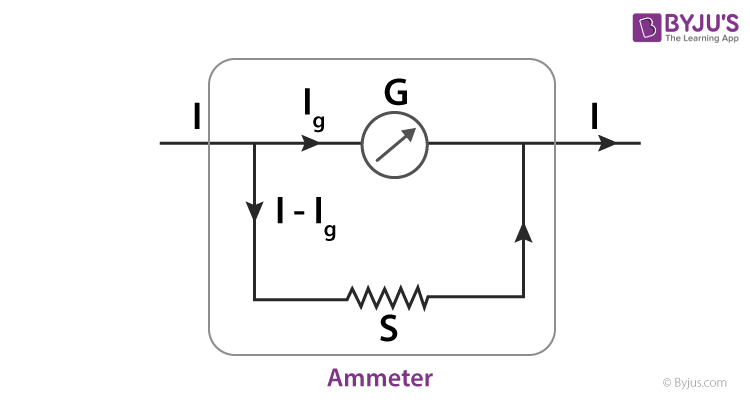# Moving Coil Galvanometer

We have discussed currents and voltages in the previous sections and in many instances, we are required to measure them. But how do we perform the measurements? We have read about voltmeter that is used to measure the voltage across two points in a circuit, or an ammeter that is used to measure the current in a circuit. A moving coil galvanometer is one such device. In this section, we will learn about a moving coil galvanometer that is used to measure the current in a circuit.

## What is Moving Coil Galvanometer

Moving coil galvanometer is defined as

The most sensitive electromagnetic device which is used for measuring and detecting small amount of electric current.

The components of moving coil galvanometer are coil, soft iron core, non-metallic frame, permanent horseshoe magnets, pivoted spring, scale, and pointer.

There are two types of moving coil galvanometer:

• Suspended coil galvanometer
• Pivoted coil galvanometer (also known as Weston galvanometer)

## Working of Moving Coil Galvanometer

When the current to be calculated is passed through the coil, the coil experiences a deflecting torque resulting in turning of the coil. The coil turns, the wire gets twisted such that restoring couple develops in the wire in opposite direction.

Let n be the number of turns of the coil, A to the area of the coil, B is the magnetic induction field, and I is the current through the coil.

The coil experiences a constant and maximum deflecting torque as the plane of the coil is parallel to the magnetic field.
$\tau d=nIAB$

Where C is the restoring couple per twist and θ is the deflection of the coil, then restoring torque is given as
$\tau r=C\theta$

The deflecting torque is equal to the restoring torque when it is in equilibrium position.
$\tau d=\tau r$ $nIAB=C\theta$ $I=\frac{C}{nAB\theta }$ $I=k\theta$

Where $k=\frac{C}{nAB}$

∴ I ∝ θ

Thus, a galvanometer is capable of indicating even small values of current in the circuit because of which it is also used to detect if any amount of current is flowing in the circuit or not, quite similar to a Wheatstone’s bridge. Here, when no current is flowing through the circuit, the pointer remains undeflected, that is, at the mean position and depending upon the direction of the current in the circuit, the pointer deflects to the right or to the left.

## Principle of Moving Coil Galvanometer

The principle behind moving coil galvanometer is that when current carrying coil is suspended in a uniform magnetic field it is acted by the torque. So it can be said that the deflection in the coil in a moving coil galvanometer is directly proportional to the current flowing through the coil.

## Construction of Moving Coil Galvanometer (Pivoted)The galvanometer consists of a coil with large number of turns insulated with copper wire on an aluminum frame.

The ends of the aluminum frame axle are inserted into two pivots so that the coil rotates about the axle. Two springs are attached to produce controlling torque and to connect the coil to the outer circuit.

The rotation of coil happens due to the magnetic field produced by the pole-pieces of a permanent strong horseshoe magnet.

The deflection is read with the help of a pointer which is attached to the coil moving on the circular scale. Zero point of the scale is at the center and has equidistant divisions. The device can measure currents up to 10-6 A.

## Sensitivity of Moving Coil Galvanometer

Sensitivity of moving coil galvanometer is defined as

The ratio of change in deflection of the galvanometer to the electric current change.

There are two types of sensitivity:

• Current sensitivity
• Voltage sensitivity

### How to increase Sensitivity of Moving Coil Galvanometer?

Following are the parameters which help in increasing sensitivity of moving coil galvanometer depends on:

• Number of turns (n) of the coil
• Area of the coil (A)
• Magnetic induction (B)
• Couple per unit twist of the suspension fibre (k)

### Limitations to increase Sensitivity of Moving Coil Galvanometer

• The resistance of the coil increases if the number of turns of the coil are increased as the length of the wire too increases.
• If the area of the coil exceeds the limit, the instrument will become bulky.
• Load on suspension increases with an increase in turns and area resulting in higher k value which reduces the sensitivity of the galvanometer.
• The weight of the apparatus depends on the strength of magnetic induction.
• The strength of the spring reduces if the couple per unit twist of the spring reduces.

## Conversion of Galvanometer into Voltmeter

A galvanometer can be converted into voltmeter by connecting a resistor with high resistance in series known as multiplier. The value of multiplier is dependent on the range requirement of the voltmeter.

Where,

• G is the resistance of galvanometer coil
• Ig is the full-scale current of the galvanometer
• R is the multiplier connected in series with galvanometer
• V is the maximum potential difference that needs to be measuredFrom the above figure, Ig (G + R) = V, hence, the R value is obtained.

## Conversion of Galvanometer into Ammeter

A galvanometer can be converted into ammeter by connecting a resistor with low resistance in parallel known as shunt. The value of shunt is dependent on the range requirement of the ammeter.Where,

• G is the resistance of galvanometer coil
• Ig is the full-scale current of the galvanometer
• S is the shunt resistance connected in parallel with galvanometer
• I is the maximum current that needs to be measured

∴ Current through shunt = I -Ig
The potential difference across G and S is:
Ig.G = (I – Ig) S, S is obtained from the equation.

Related Article:

## Advantages of Moving Coil Galvanometer

• Strong magnetic field cannot affect the moving coil galvanometer.
• The ratio of torque to weight is high.
• The scales used are uniform.
• The results obtained are reliable and accurate.

## Disadvantages of Moving Coil Galvanometer

• Change in restoring torque is a result of change in temperature.
• It is not easy to change restoring torque.
• Helical restoring spring might get damaged due to severe stress.
• Moving coil galvanometer can be used only for the measurement of direct current.